# Ratios Unit 1 Lesson 1 Math 6 Ratios

• Slides: 18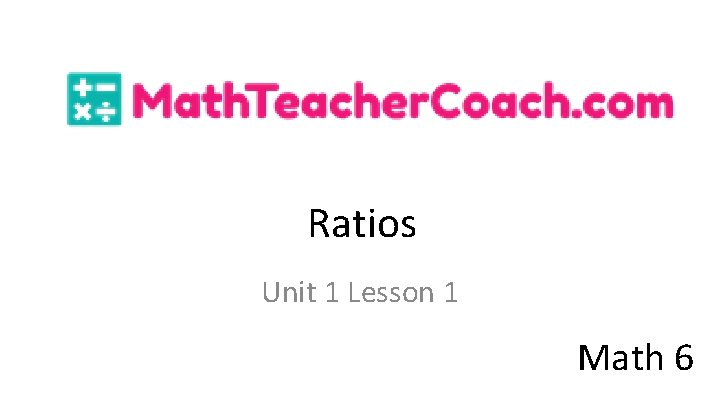Ratios Unit 1 Lesson 1 Math 6Ratios Students will be able to: Determine the comparison between or relationship of two things using ratio. Solve word problems involving ratio.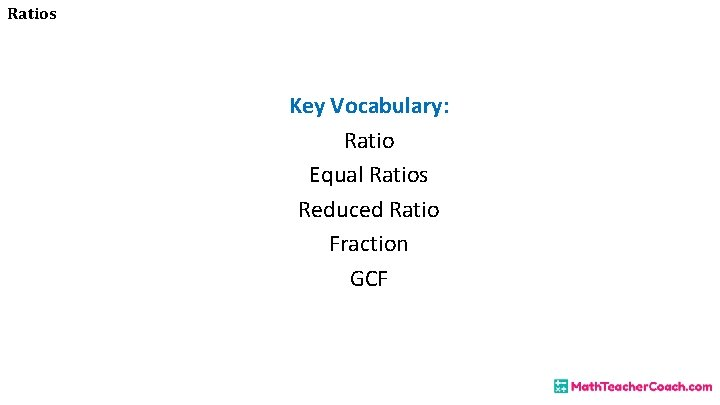Ratios Key Vocabulary: Ratio Equal Ratios Reduced Ratio Fraction GCFRatios Ratio is a comparison between, or a relationship of two things. Example: There is 1 ice cream cone to 3 cookies.Ratios Example: There are 4 boys to 2 girls.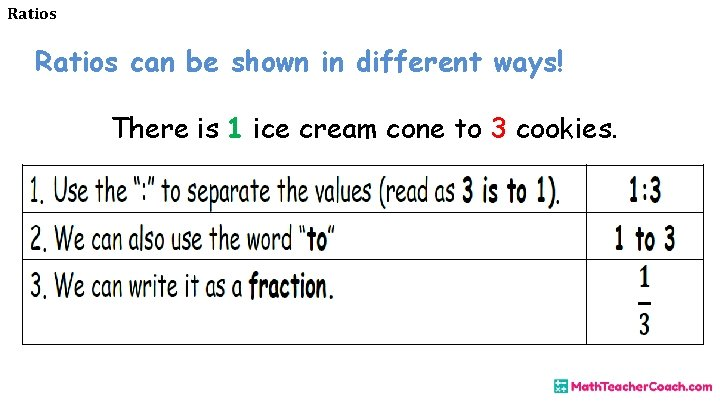Ratios can be shown in different ways! There is 1 ice cream cone to 3 cookies.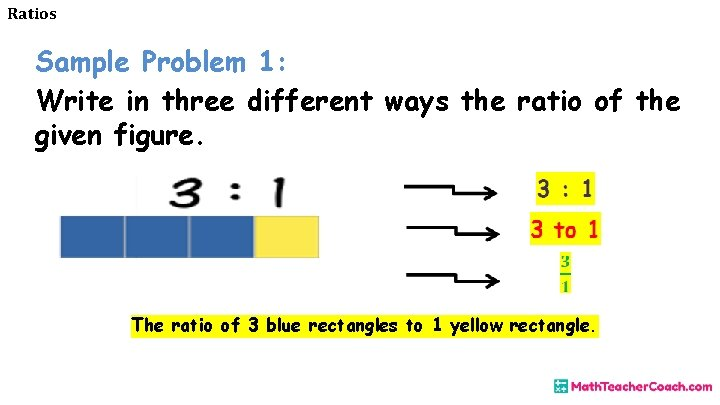Ratios Sample Problem 1: Write in three different ways the ratio of the given figure. The ratio of 3 blue rectangles to 1 yellow rectangle.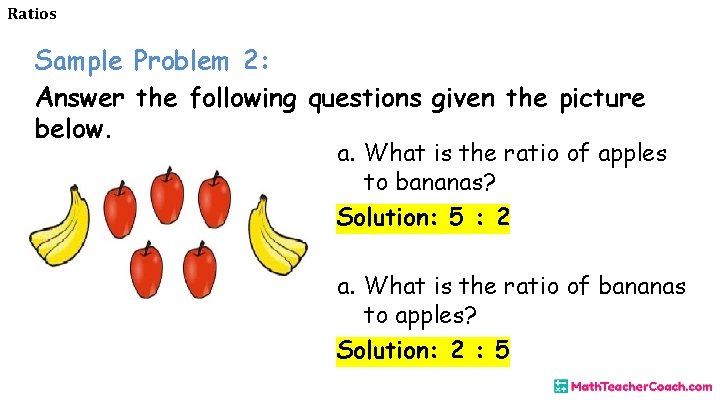Ratios Sample Problem 2: Answer the following questions given the picture below. a. What is the ratio of apples to bananas? Solution: 5 : 2 a. What is the ratio of bananas to apples? Solution: 2 : 5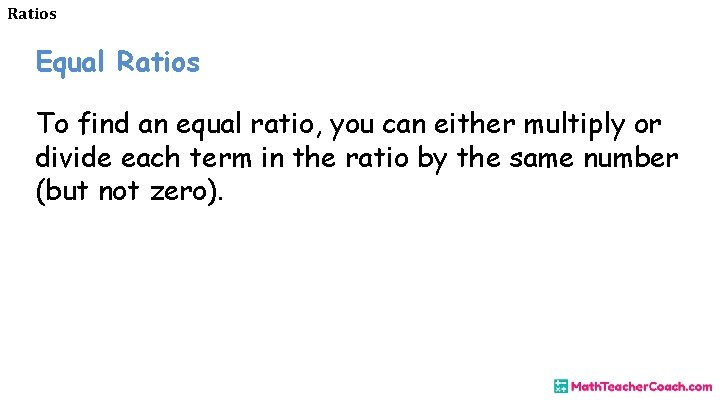Ratios Equal Ratios To find an equal ratio, you can either multiply or divide each term in the ratio by the same number (but not zero).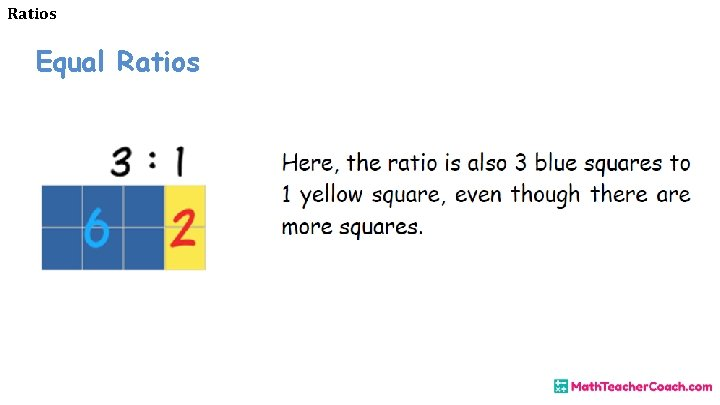Ratios Equal Ratios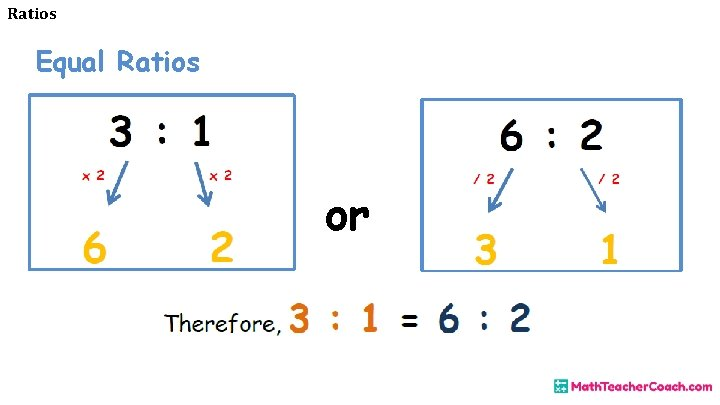Ratios Equal Ratios orRatios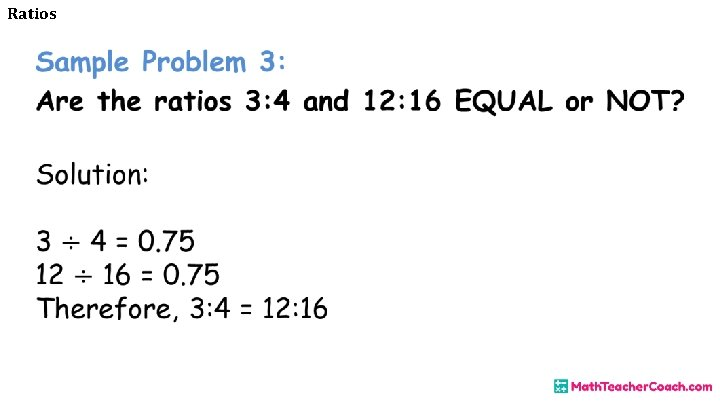RatiosRatios Reducing ratios is similar to reducing a fraction in lowest terms since ratios can be expressed as fractions. Example: Reduce 12: 16 in lowest terms. Step 1: Find the GCF of the numbers in the ratio. GCF is 4Ratios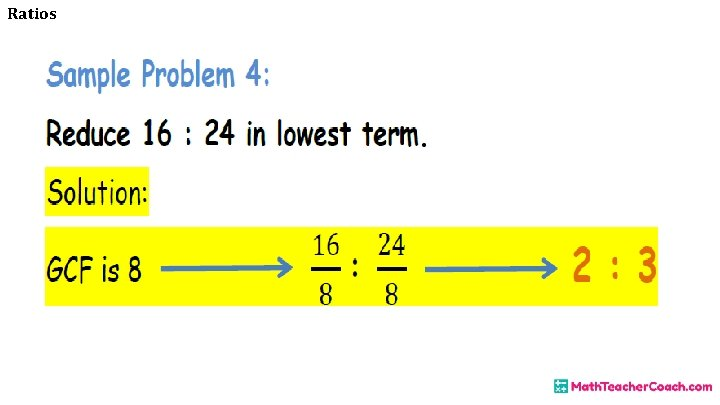Ratios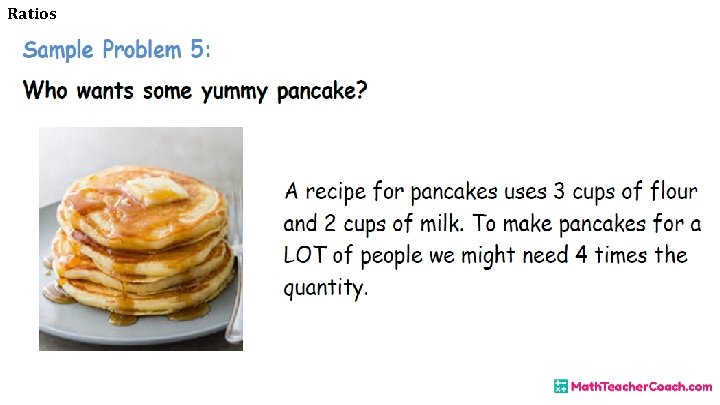Ratios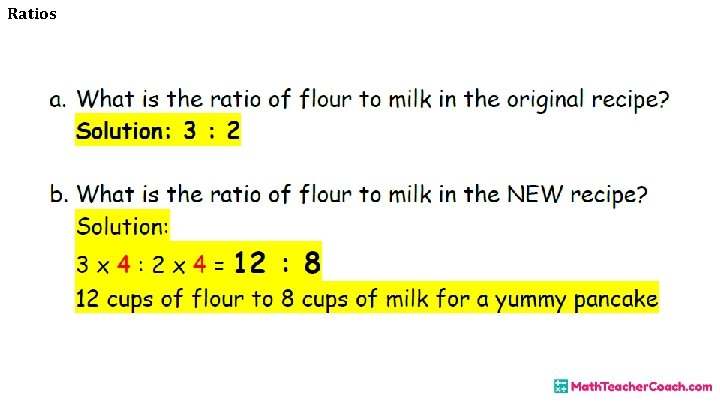Ratios